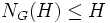# GAP:IsSelfNormalizing

Jump to: navigation, search

## Definition

### Function type

The function takes as input two arguments, both of which are groups, and outputs a boolean variable.

### Behavior

The function behaves as follows:

• If the two groups are contained in a common big group and the normalizer of the second group in the first group is also contained in the second group, the function returns true. In other words, if the groups are$G,H$ and$N_G(H) \le H$, the function returns true.
• If the two groups are in a common big group, but the normalizer of the second group in the first group is not contained in the second group, the function returns false.
• If the two subgroups are not subgroups of a common big group, GAP returns a NoMethodFound error.

### Typical usage

The typical usage is as follows:

`IsSelfNormalizing(group,subgroup);`

This returns true if and only if the subgroup is a self-normalizing subgroup of the whole group.

## Method

### Code

```IsSelfCentralizing := function(G,H)
return IsSubgroup(H, Normalizer(G,H));
end;;```